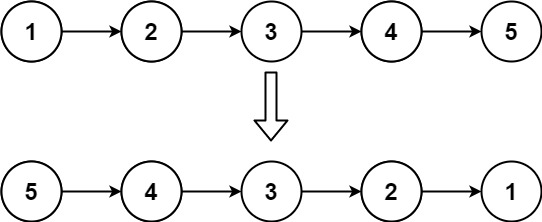# Week 2 | Grind 169 | Blind 75

Posted on Dec 25, 2020

## 1. Ransom Note

Given two strings ransomNote and magazine, return true if ransomNote can be constructed by using the letters from magazine and false otherwise.

Each letter in magazine can only be used once in ransomNote.

``````bool canConstruct(string ransomNote, string magazine) {

int hash = {0};

for(char ch:magazine)
hash[ch-'a']++;

for(char ch:ransomNote)
if(hash[ch-'a'] <= 0)
return false;
hash[ch-'a']--;

return true;
}
``````

## 2. Ransom Note

You are climbing a staircase. It takes n steps to reach the top.

Each time you can either climb 1 or 2 steps. In how many distinct ways can you climb to the top?

``````int climbStairs(int n, int rem = 0) {
vector<int> dp(n+1);
dp=dp=1;
for(int i=2;i<=n;i++)
dp[i]=dp[i-1]+dp[i-2];
return dp[n];
}
``````

## 3. Longest Palindrome

Given a string s which consists of lowercase or uppercase letters, return the length of the longest palindrome that can be built with those letters.

Letters are case sensitive, for example, `Aa` is not considered a palindrome here.

``````    int longestPalindrome(string s) {
int ans(0), n = s.size();
bool inMiddle = false;
if(n < 2) return 1;

unordered_map<char, int> mp;

for(char ch:s)
mp[ch]++;

for(auto e:mp){
ans += e.second/2;

if(!inMiddle and e.second % 2)
inMiddle = true;
}

if(inMiddle)
return (ans*2)+1;

return ans*2;
}
``````

Given the head of a singly linked list, reverse the list, and return the reversed list.``````ListNode* reverseList(ListNode* head) {
ListNode *prev = NULL;

}

return prev;
}
``````

Given two binary strings `a` and `b`, return their sum as a binary string.

``````string addBinary(string a, string b) {
int i = a.length()-1, j = b.length()-1, carry(0);
string ans = "";

while(i>=0 || j>=0){

if(i>=0)
carry += a[i--] - '0';

if(j>=0)
carry += b[j--] - '0';

ans += (carry%2 + '0');
carry = carry/2;
}

if(carry) ans += (carry%2 + '0');

reverse(ans.begin(),ans.end());
return ans;
}
``````

## 9. Depth of Binary Tree

Given the root of a binary tree, return its maximum depth.

A binary tree’s maximum depth is the number of nodes along the longest path from the root node down to the farthest leaf node.

``````int maxDepth(TreeNode* root) {
return root ? max(maxDepth(root->left), maxDepth(root->right))+1 : 0;
}
``````

## 8. Diameter of Binary Tree

Given the `root` of a binary tree, return the length of the `diameter` of the tree.

The `diameter` of a binary tree is the length of the longest path between any two nodes in a tree. This path may or may not pass through the `root`.

The length of a path between two nodes is represented by the number of edges between them.

``````    int height = 0;

int dfs(TreeNode* root){

if(!root) return 0;

int left = dfs(root->left);
int right = dfs(root->right);

if(left + right > height)
height = left + right;
return max(left, right) + 1;
}

int diameterOfBinaryTree(TreeNode* root) {
dfs(root);

return height;
}
``````

## 9. Middle of the Linked List

If there are two middle nodes, return the second middle node.### Two Pass

``````    ListNode* middleNode(ListNode* head) {
int len = 0;
while(curr != NULL)
{
len++;
curr = curr->next;
}

len /=2;

while(len--)
}
``````

### Single Pass

``````ListNode* middleNode(ListNode* head) {

while(fast and fast->next){
fast = fast->next->next;
slow = slow->next;
}

return slow;

}
``````

## 10. Depth of Binary Tree

Given the root of a binary tree, return its maximum depth.

A binary tree’s maximum depth is the number of nodes along the longest path from the root node down to the farthest leaf node.

``````int maxDepth(TreeNode* root) {
return root ? max(maxDepth(root->left), maxDepth(root->right))+1 : 0;
}
``````

## 10. Depth of Binary Tree

Given an integer array nums, return \$true\$ if any value appears at least twice in the array, and return \$false\$ if every element is distinct.

``````bool containsDuplicate(vector<int>& nums) {

unordered_map<int, int> mp;

for(int e:nums){
if(mp[e])
return true;
mp[e]++;
}

return false;
}
``````

## 9. Middle of the Linked List

If there are two middle nodes, return the second middle node.### O(nlogn)

``````int getSum(int n){
int ans = 0;

while(n){
if(n%2) ans++;
n = n/2;
}

return ans;
}

vector<int> countBits(int n) {
vector<int> ans;

for(int i=0; i<=n; i++)
ans.push_back(getSum(i));

return ans;
}
``````

### O(n)

``````vector<int> countBits(int n) {

vector<int> dp(n+1);
dp=0;

for(int i=1; i<n+1;i++)
dp[i] = dp[i/2] + i%2;

return dp;
}
``````